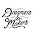## Multiple Choice Questions Based on Power Electronics and Drives

 When a line commutated converter operates in the inverter mode
(a) It draws both real and reactive power from the AC supply
(b) It delivers both real and reactive power to the AC supply
(c) It delivers real power to the AC supply
(d) It draws reactive power from the AC supply

Answer: C

 A chopper operating at a fixed frequency is feeding an RL load. As the duty ratio of the chopper is increased from 25% to 75%, the ripple in the load current is
(a) Remains constant
(b) Decreases, reaches a minimum at 50% duty ratio and then increases
(c) Increases, reaches a maximum at 50% duty ratio and then decreases
(d) Keeps on increasing as the duty ratio is increased

Answer: A

 To turn off a SCR, the reverse bias should be applied for a period ....... the turn-off time of the SCR
(a) Equal to
(b) Longer than
(c) Less than
(d) Irrespective of

Answer: B

 In class A and class B commutation the resonating circuit has to be
(a) Over damped
(b) Critically damped
(c) Under damped
(d) Negatively damped

Answer: C

 In phase controlled rectification power factor (PF)
(a) Remains unaffected
(b) Improves with increase of firing angle α
(c) Deteriorates with increase of α
(d) Is unrelated to α

Answer: C

 Comparing with the full wave rectifier using two diodes, the four diode bridge rectifier has the dominant advantage of
(a) Higher current carrying
(b) Lower peak inverse voltage requirement
(c) Lower ripple factor
(d) Higher efficiency

Answer: B

 A SCR is rated at 75A peak, 20A average. The greatest possible delay in the trigger angle if the dc is at rated value is
(a) 47.5°
(b) 30° to 45°
(c) 74.5°
(d) 137°

Answer: B

 The applied sine voltage to a SCR is VM=200V and R=10Ohm. If the gate trigger lags the ac supply by 120°, the average load current is
(a) 15/π A
(b) 5/π A
(c) -5/π A
(d) -15/π A

Answer: A

 A sine voltage of 200Vrms, 50Hz is applied to an SCR through 100ohm resistor. The firing angle is 60°. Consider no voltage drop. The output voltage in rms is
(a) 89.7 V
(b) 126.7 V
(c) 166.7 V
(d) 200√2 V

Answer: B

 A 100VDC is applied to the inductive load through a SCR. The SCR's specified latching current is 100mA. The minimum required width of gating pulse to turn on the SCR is
(a) 100μS
(b) 100S
(c) 1mS
(d) 50μS

Answer: A

 A cycloconverter is a
(a) Frequency changer from higher to lower frequency with one-state conversion
(b) Frequency changer from higher to lower frequency with two-stage conversion
(c) Frequency changer from lower to higher frequency with one-stage conversion
(d) Either a or c

Answer: D
 The cycloconverter require natural or forced commutation as under
(a) Natural commutationin bothstep-up and step down cycloconverter
(b) Forced commutation in both step-up and step-down cycloconverter
(c) Forced commutation in step-up cycloconverter
(d) Forced commutation in step-down cycloconverter

Answer: C
 In synchronized UJT triggering of an SCR, voltage VC across capacitor reaches UJT threshold thrice in each half cycle so that there are three firing pulses during each half cycle. The firing angle of the SCR can be controlled
(a) Once in each half cycle
(b) Thrice in each half cycle
(c) Twice in each half cycle
(d) Four times in each half cycle
(e) None of the above

Answer: A
 In a GTO, anode current begins to fall when gate current
(a) Is negative peak at time t=0
(b) Is negative peak at t = storage period tS
(c) Just begins to become negative at t = 0
(d) Is negative peak at t = (tS + fall time)

Answer: B
 The SCR can be turned on by
(a) Applying anode voltage at a sufficiently fast rate
(b) Applying sufficiently large anode voltage
(c) Increasing the temperature of SCR to a sufficiently large value
(d) Applying sufficiently large gate current
OPTIONS:
1) A, B
2) C, D
3) B, C
4) A, B, C, D
5) None of the above options

Answer: 4

Solution Hint:
 VL= V[(Ton/(Ton+Toff)]
=V. f .Ton
There are two ways to vary VL. Either by varying f or Ton. Here f is fixed and Ton is     varied. As the frequency is kept constant ripple remains constant.

 The PIV of diodes used in the full wave rectifier using two diodes is 2 times that of  the four diode bridge rectifier

 RMS Current/ Average Current = Form Factor

 Idc = [Vm/2πR](1+cosα)
α = delay angle

 a= dv/dt triggering, b = forward voltage triggering, c= temperature triggering, d = gate triggering. So all are correct
7:09 AM

#### 5 comments:

1.nice quastions

2.3.4.i want know from where to taken for 7 and 9 question. source of book.please help

5.Solution Hint for No.10